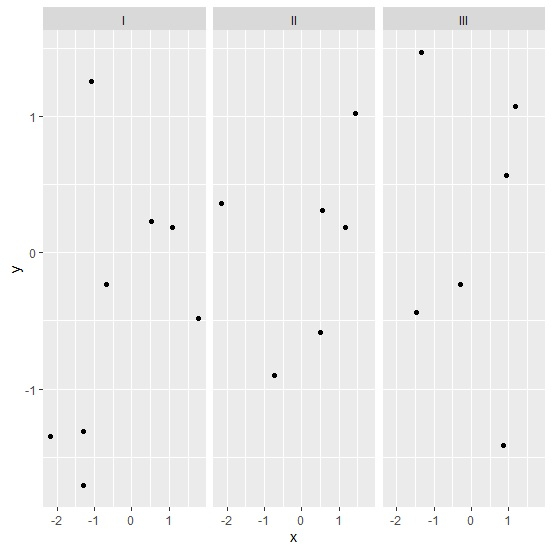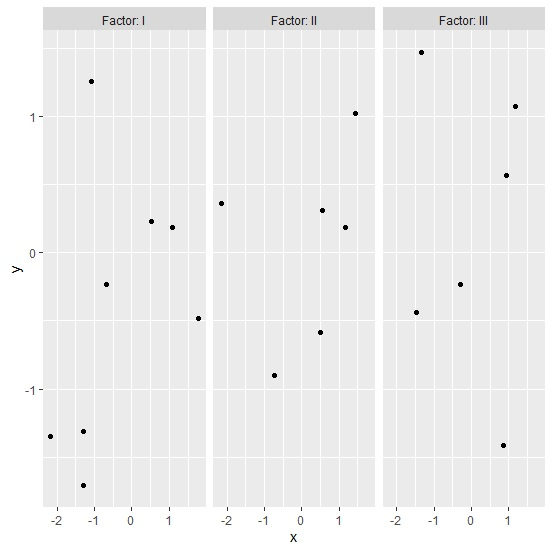# How to display categorical column name in facet grid in R?

To display categorical column name in facet grid, we can use the following steps −

• First of all, creating data frame.
• Creating the chart with facets and labeller function to display categorical column name

## Create the data frame

Let's create a data frame as shown below −

Live Demo

x<-rnorm(20)
y<-rnorm(20)
Factor<-sample(c("I","II","III"),20,replace=TRUE)
df<-data.frame(x,y,Factor)
df

On executing, the above script generates the below output(this output will vary on your system due to randomization) −

       x         y     Factor
1  -1.2961065 -1.3092987 I
2   0.5337357  0.2291017 I
3   1.7851655 -0.4845764 I
4   1.0809707  0.1879267 I
5  -0.7360667 -0.8993411 II
6  -2.1703410 -1.3451734 I
7   1.1761357  0.1834939 II
8   1.4432419  1.0218972 II
9  -0.2989334 -0.2337353 III
10  0.5419797  0.3144817 II
11 -1.2864239 -1.7106241 I
12 -1.0866636  1.2598880 I
13 -0.6756130 -0.2291350 I
14 -1.4876827 -0.4388541 III
15 -2.1607323 0.3629047 II
16 0.9352823 0.5710842 III
17 1.1757290 1.0738887 III
18 0.5019893 -0.5860239 II
19 -1.3477106 1.4709259 III
20 0.8654047 -1.4147550 III

Create a point chart for groups displayed in facets −

library(ggplot2)
x<-rnorm(20)
y<-rnorm(20)
Factor<-sample(c("I","II","III"),20,replace=TRUE)
df<-data.frame(x,y,Factor)
ggplot(df,aes(x,y))+geom_point()+facet_grid(~Factor)

### Output## Creating facetted chart with categorical column name in facet grid

Use labeller function of ggplot2 package to create the point chart with facets having Factor column values displayed in facets −

x<-rnorm(20)
y<-rnorm(20)
Factor<-sample(c("I","II","III"),20,replace=TRUE)
df<-data.frame(x,y,Factor)
ggplot(df,aes(x,y))+geom_point()+facet_grid(~Factor,labeller=labeller(.rows=label_both,
.cols=label_both))

### Output